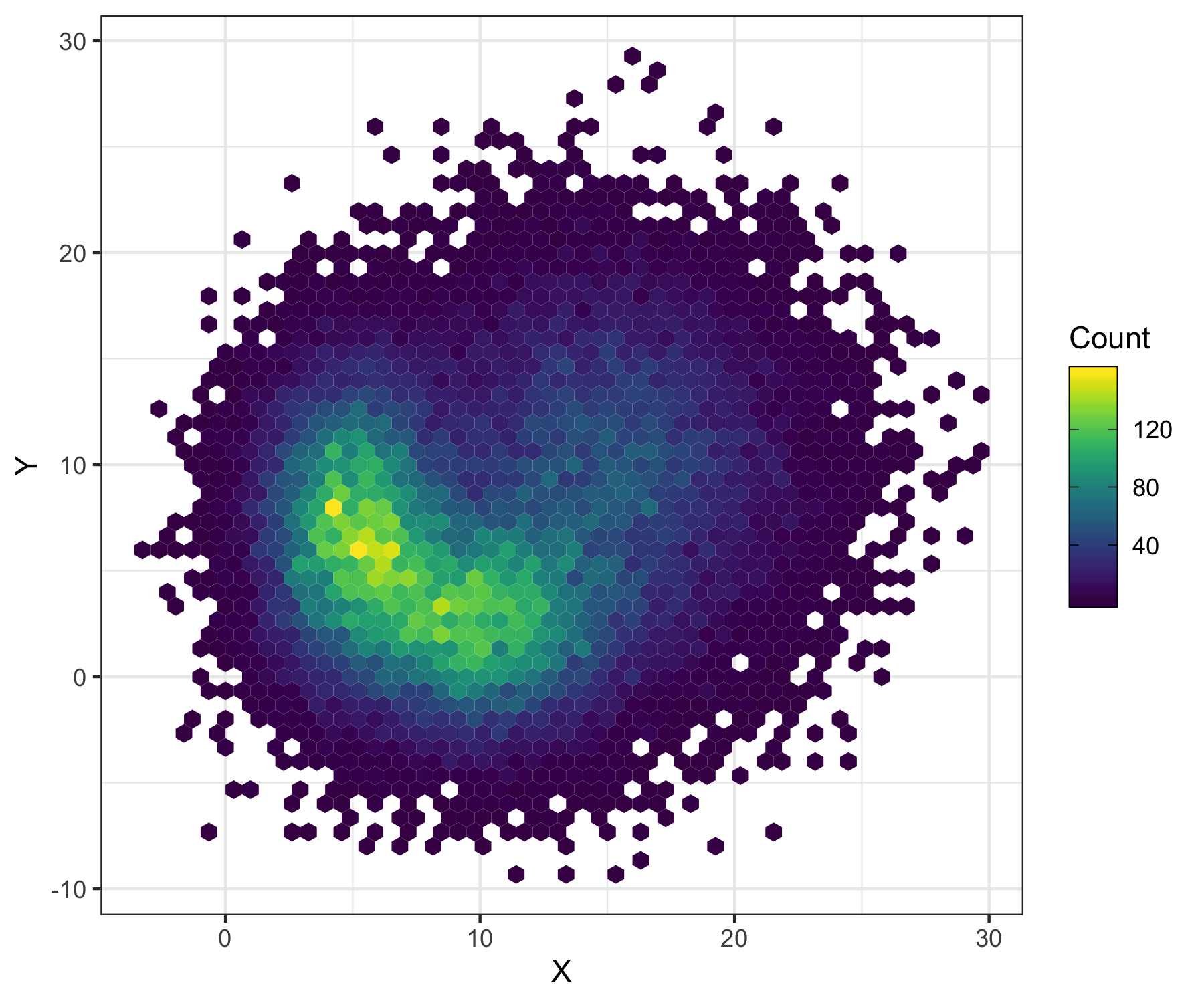# Intensity plot 2

Category: intensity``````library(tidyverse)
library(viridis)

n <- 15000

data <- bind_rows(
tibble(x = rnorm(n, 5, 2), y = rnorm(n, 7.5, 4)),
tibble(x = rnorm(n, 10, 3), y = rnorm(n, 2.5, 3)),
tibble(x = rnorm(n, 15, 4), y = rnorm(n, 10, 5))
)

p <- ggplot(data) +
geom_hex(aes(x, y), bins = 50) +
theme_bw() +
labs(x = 'X', y = 'Y') +
scale_fill_viridis(
name = 'Count',
guide = guide_colorbar(frame.colour = 'black', ticks.colour = 'black')
) +
theme(legend.position = 'right')
ggsave('2.png', p, height = 5, width = 6)
``````